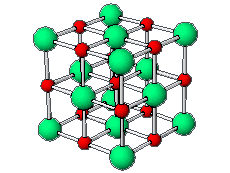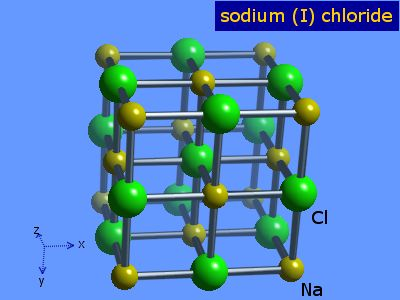# Question #2d908

Sep 19, 2015#### Explanation:

You may want to draw the bonds between them. Just remember that the cation will be attracted to the anion.

Jan 15, 2016

It is difficult to draw the lattice structures of ionic compounds without a computer.

#### Explanation:

It is more important that you be able to recognize the unit cells in the lattice structures.

The positive and negative ions are often in a cubic array. Here are two examples.

$\text{CsCl}$, a simple cubic structure

The ${\text{Cl}}^{-}$ ions are the blue atoms at the corners of the cube.The ${\text{Cs}}^{+}$ ions are at the centre of the cube.

The unit cell extends through the whole crystal.

This animation shows the $\text{CsCl}$ lattice, the $\text{Cs}$ sublattice in blue, and the $\text{Cl}$ sublattice in brown.$\text{NaCl}$, a face centred cube

The green ${\text{Cl}}^{-}$ ions occupy each corner and the centre of each face of the cube.In the picture below, the ${\text{Na}}^{+}$ ions also occupy a face-centred cubic unit cell.These unit cells extend through the whole crystal.